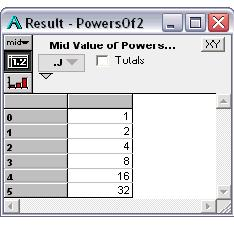# Index..Do

The construct, Index i := seqExpr defines an index local to the definition in which it is used. The expression seqExpr may be a sequence, literal list, or other expression that generates an unindexed array, as used to define a global index. For example:

Variable PowersOf2
Definition: Index J := 0..5;
2^J


The new Variable PowersOf2 is an array of powers of two, indexed by the local index J, with values from 0 to 5:

PowersOf2 →# Dot Operator: A.I

The dot operator in A.I lets you access a local index I via an array A that it dimensions. If a local index identifies a dimension of an array that becomes the value of a global Variable, it may persist long after valuation of the expression—unlike other local Variables which disappear after the expression is evaluated.

Even though local index J has no global identifier, you can access it via its parent Variable with the dot operator, ’.’, for example:

PowersOf2.J → [0,1,2,3,4,5]

When using the subscript operation on a Variable with a local index, you need to include the ’.’ operator, but do not need to repeat the name of the Variable:

PowersOf2[.J=5] → 32

Any other Variables depending on PowersOf2 may inherit J as a local index—for example:

Variable P2
Definition: PowersOf2/2

P2[.J=5] → 16

# Examples using a local index

In this example, MatSqr is a user-defined function that returns the square of a matrix—i.e., A x A, where A is the transpose of A. The result is a square matrix. Rather than require a third index as a parameter, MatSqr creates the local index, I2, as a copy of index I.

Function MatSqr(a: ArrayType; i,j: IndexType)
Definition:
Index I2:=CopyIndex(i);
Sum(a*a[i=I2], j)


The local Variable, I2, in MatSqr is not within lexical scope in the definition of Z, so we must use the dot operator ’.’ to access this dimension. We underline the dot operator for clarity:

Variable Z
Definition:
Var XX := MatSqr(X, Rows, Cols);
Sum(XX * Y[I=XX.I2], XX.I2)


# Syntactic Variations

Index I := seqExpr Do bodyExpr


or

Index I := seqExpr;
expr;
expr;
...


To control the index name, separate from the local variable name:

Index I / nameExpr := seqExpr Do bodyExpr